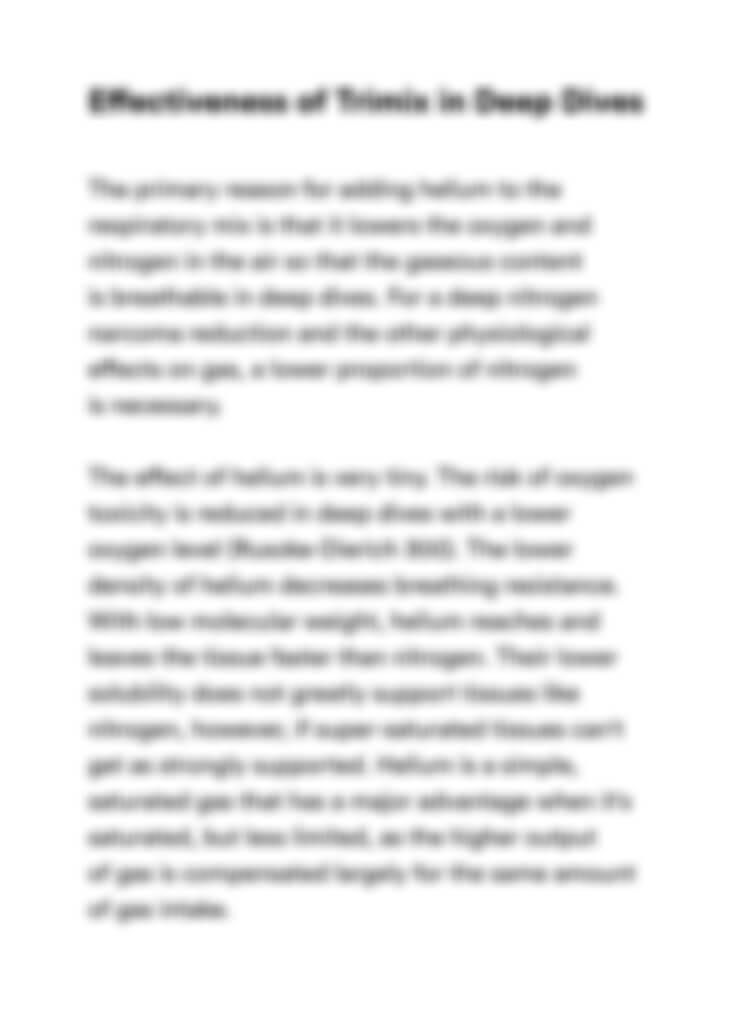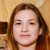## Document preview:

MEAN Mean= the total sum divided by the count = MEDIAN Arrange the numbers in ascending order 125106, 139106, 143209, 145809, 63659, 186731, 290596, 350634, 440584, 1172246 Median value= (5th term+6th term)/2 = The median best represents the data since is a more skewed the distribution (the difference between the median and mean is great), and the greater emphasis should be placed on using the median as opposed to the mean 30. MODE Mode= number which appears most often in a set of numbers The mode for this data is 55, 58, 60 and 62 RANGE Range= difference between the highest and lowest values in the set = MEAN Mean= the total sum divided by the count MEDIAN Arrange the numbers in ascending order 44, 46, 48, 49, 50, 51, 52, 54, 55, 55, 57, 58 , 58, 59, 60, 60, 62, 62, 63, 64, 65, 67, 69, 73, 75 The median for this data is the 13th (middle) number when the data is arranged in ascending order which is 58 The mean best measure of central tendency in this case because it is the m...

\$20
Enter valid email

Homework mathLitTeacher1
4.5 (166 reviews)

4 in Law

241 completed projects

## This might be interestingDescriptive Statistics ProjectLitTeacher1
4.5